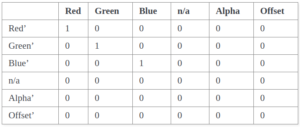Related Articles

# Python – color_matrix() function in Wand

• Last Updated : 08 May, 2020

color_matrix() method allows you to recalculate color values by applying a matrix transform. A matrix can be up to a 6×6 grid where each column maps to a color channel to reference, and each row represents a color channel to effect.red, green, blue, n/a, alpha, and a constant (a.k.a offset) describe the corresponding rows and columns.Syntax :

```wand.image.color_matrix(matrix)
```

Parameters :

ParameterInput TypeDescription
matrixcollections.abc.Sequence2d list of doubles.

Source Image :Example 1:

 `# Import Image from wand.image module``from` `wand.image ``import` `Image`` ` `# Read image using Image function``with Image(filename ``=``"koala.jpeg"``) as img:``    ``matrix ``=` `[[``0``, ``0``, ``1``],``              ``[``0``, ``1``, ``0``],``              ``[``1``, ``0``, ``0``]]``    ``# Recalculate color using color_matrix() method``    ``img.color_matrix(matrix)``    ``img.save(filename ``=``"cm_koala.jpeg"``)`

Output:Example 2:

 `# Import Image from wand.image module``from` `wand.image ``import` `Image`` ` `# Read image using Image function``with Image(filename ``=``"koala.jpeg"``) as img:``    ``matrix ``=` `[[``0``, ``1``, ``0``],``              ``[``1``, ``0``, ``0``],``              ``[``0``, ``0``, ``1``]]``    ``# Recalculate color using color_matrix() method``    ``img.color_matrix(matrix)``    ``img.save(filename ``=``"cm_koala2.jpeg"``)`

Output:Attention geek! Strengthen your foundations with the Python Programming Foundation Course and learn the basics.

To begin with, your interview preparations Enhance your Data Structures concepts with the Python DS Course. And to begin with your Machine Learning Journey, join the Machine Learning – Basic Level Course

My Personal Notes arrow_drop_up